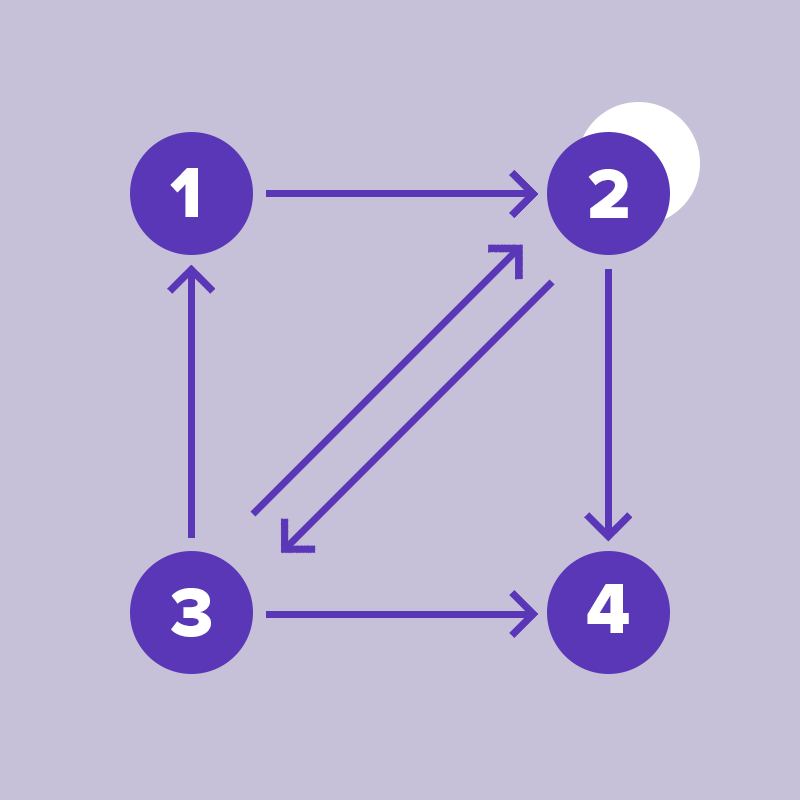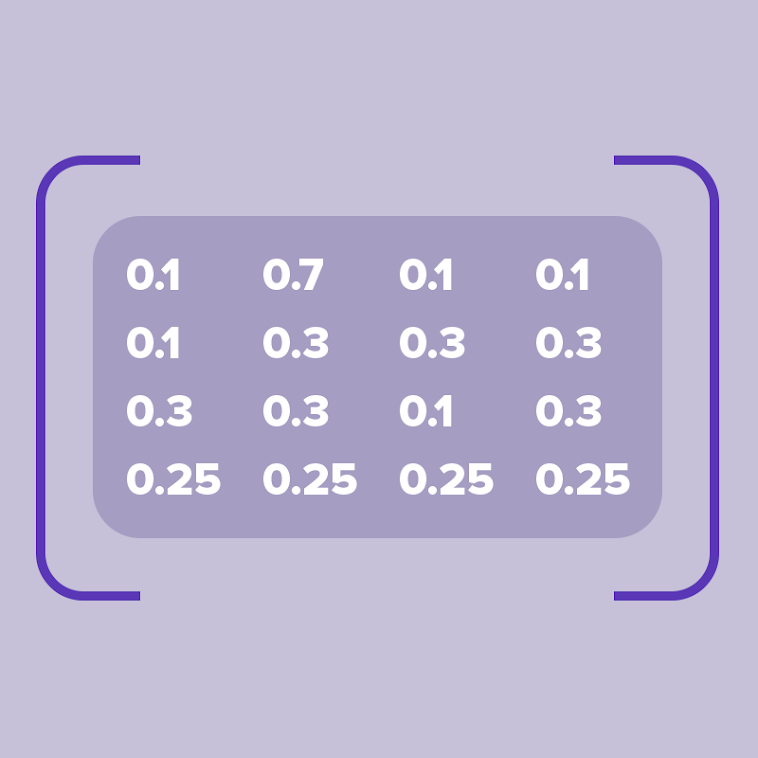Extracting information from data has the power to change all aspects of life. The is an application which uses different protocols for information sharing and communication. With such a vast majority of resources and data present on the world wide , it becomes essential to dig out useful information in a cost-efficient manner. is the process of discovering knowledge from the data present on the internet. One of the building blocks of the internet are hyperlinks which connect different pages or resources. Therefore is a method that we can use to find out the popularity of a web page. analysis uses the theory that if a web page has a higher probability of being visited then it might have a higher probability of being popular and thereby it can be more relevant.

## Link analysis

Links are an essential element of almost all web pages. In the html code, these links are placed within the href attribute of an anchor tag. Consider the following graph where each node denotes a web page and the directed edges denote a link between two pages. The edge from node 1 to 2 indicates that the html code of node 1 has an anchor tag whose href attribute establishes a link with node 2 as the destination.The link between page 2 and 3 is bidirectional and page 2 has a self link. The link matrix for the above graph is as follows where 1 indicates the presence of a link with source page as the row number and destination page as the column number. 0 indicates the absence of a link.The probability to navigate from one page (denoted by the row number) to another page(denoted by the column number) is shown in the transition probability matrix below.

These probability values are obtained by counting the number of ones across each row of the link matrix and replacing the value 1 with the value of 1/N. Thus the sum of probabilities across a row adds up to 1.

Dead End and Teleporting

Given a random start page, if a web surfer decides to use only the outgoing links to navigate to other pages then in case of a random walk, the surfer can get stuck at a dead end. This happens upon reaching a page with no outgoing links (Node 4 in the above example). These dead ends make it difficult to compute a page’s rank as the probability of the page’s long term visit rate is not defined. Teleporting is used to get out of a dead end and go to any other page with equal probability.

## Transition probability

Since the above graph has 4 nodes (N = 4). Using teleportation, the probability of jumping out from the dead end at node 4 is ¼ or 0.25. If the probability of teleportation () is 0.4 then

/N = 0.1. Adding 0.1 to all zeros of the non-dead end rows and subtracting appropriate values to make sure that the probability across each adds up to 1 gives the following transition probability matrix for the above web graph.## Transition probability matrix with teleporting

This transition probability matrix indicates how likely a web surfer whose only option is to follow the links on the current page or teleport away if there are no links can move forward.

## Conclusion

Link analysis is one of the many methods used for web mining. It is an important component of the PageRank algorithm. There are several components of web ranking like PageRank, anchor text, proximity etc. The awareness about some of those can be a starting point to help you explore and implement methods that can make your web pages more popular.

Source link https://www.codingdojo.com/blog/web-mining-using-link-analysis/

SHARE